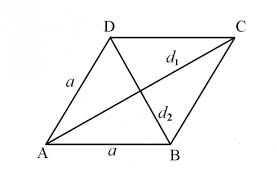## Rhombus Area & Perimeter Calculator

 Rhombus Area Using Trigonometry calculator Length of Rhombus (A) Enter Angle
 Result: Area of Rhombus Square Unit Perimeter of Rhombus Unit

 Rhombus Area using Diagonal Method Calculator Diagonal Length D1= D2   = Result: Area of Rhombus Square Unit

Rhombus is a shape with four angles which are not 90-degrees and four equal sides.

Rhombus Calculator to find out the Rhombus Perimeter and Area of the given values of Angle and Length.

The Area and Perimeter of rhombus can also be calculated by product of two diagonal lengths D1 and D2.

Rhombus### Trigonometry Method Formulas

Rhombus Area (Trigonometry) = A2

### Diagonal Method formulas

Rhombus Area (Diagonal method)= (D1 x D2)/2

Rhombus Perimeter = 4A

Thinkcalculator.com provides you helpful and handy calculator resources.